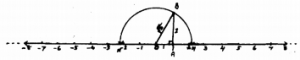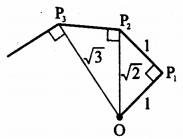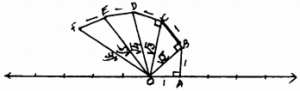# KSEEB Solutions for Class 9 Maths Chapter 1 Number Systems Ex 1.2

KSEEB Solutions for Class 9 Maths Chapter 1 Number Systems Ex 1.2 are part of KSEEB Solutions for Class 9 Maths. Here we have given Karnataka Board Class 9 Maths Chapter 1 Number Systems Exercise 1.2.

## Karnataka Board Class 9 Maths Chapter 1 Number Systems Ex 1.2

Question 1.
State whether the following statements are true or false. Justify your answers.
(i) Every irrational number is a real number.
True. Because set of real numbers contain both rational and irrational number.

(ii) Every point on the number line is of the form $$\sqrt{\mathrm{m}}$$. where’m’ is a natural number.
False. Value of $$\sqrt{\mathrm{m}}$$ is not netagive number.

(iii) Every real number is an irrational number.
False. Because set of real numbers contain both rational and irrational numbers. But 2 is a rational number but not irrational number.

Question 2.
Are the square roots of all positive integers irrational? If not, give an example of the square root of a number that is a rationed number.
Square root of all positive integers is not an irrational number.
E.g. $$\sqrt{\mathrm{4}}$$ = 2 Rational number.
$$\sqrt{\mathrm{9}}$$ = 3 Rational number.

Question 3.
Show how $$\sqrt{\mathrm{5}}$$ can be represented on the number line.
$$\sqrt{\mathrm{5}}$$ can be represented on number line:In the Right angled ∆OAB ∠OAB = 90°.
OA = 1 cm, AB = 2 cm., then
As per Pythagoras theorem,
OB2 =OA2 + AB2
= (1)2 + (2)2
= 1 + 4
OB2 = 5
∴ OB = $$\sqrt{\mathrm{5}}$$
If we draw semicircles with radius OB with ‘O’ as centre, value of $$\sqrt{\mathrm{5}}$$ on number line
$$\sqrt{\mathrm{5}}$$ = OM = +2.3
and $$\sqrt{\mathrm{5}}$$ = ON = -2.3 (accurately).

Question 4.
Classroom activity (Constructing the ‘square root spiral’): Take a large sheet of paper and construct the ‘square root spiral’ in the following fashion.Start with a point O and draw a line segment OP1 of unit length. Draw a line segment P1P22 perpendicular to OP1 of unit length (see fig.). Now draw a line segment P2P3 perpendicular to OP2. Then draw a line segment P3P4 perpendicular to OP3. Continuing in this manner, you can get the line segment Pn-1Pn by drawing a line segment of unit length perpendicular to OPn-1. In this manner, you will have created the points P2, P3, …………….. pn, …………… and joined them to create a beautiful spiral depicting $$\sqrt{2} \cdot \sqrt{3}, \sqrt{4}, \dots \dots$$∴OB = $$\sqrt{2}$$